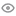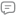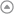New update is available. Click here to update.
My StatsEXP gained10472Level
7 (Expert)
Community statsDiscussions
01
Know more273
Total problems solved
226
Easy
40
Moderate
7
Hard
0
NinjaCurrent streak:

0 days

Longest streak:

45 days

Less

More

Achievements6
Ronin
Topics
Sorting
Math
+ 4 more1
Samurai
Topics
Arrays
Discussions
O(1) solution using Bit Manipulation
Interview problems

First we will XOR all the elements from the range [1,n] then using this approach, we have to find xor of elements from the range [1, L – 1] and from the range [1, R] and then xor the respective answers again to get the xor of the elements from the range [L, R]. This is because every element from the range [1, L – 1] will get XORed twice in the result resulting in a 0 which when XORed with the elements of the range [L, R] will give the result.

code:

//XOR elements in range[1,N]

int XOR(int n) {

int mod = n % 4;

//if n is a multiple of 4

if(mod == 0)

return n;

//if n%4 gives reaminder 1

else if(mod == 1)

return 1;

//if n%4 gives remainder 2

else if(mod == 2)

return n+1;

//if n%4 gives remainder 3

else if(mod == 3)

return 0;

}

int findXOR(int L, int R){

return (XOR(L-1) ^ XOR(R));

}

Md Tanveer Ansari
Published On 08-Oct-2023409 views0 repliesWe can do it in O(Logn) time using bitwise operators.
Interview problems

The idea is based on the following fact.

square(n) = 0 if n == 0

if n is even     square(n) = 4*square(n/2)

if n is odd     square(n) = 4*square(floor(n/2)) + 4*floor(n/2) + 1

Examples :

square(6) = 4*square(3) = 36

square(3) = 4*(square(1)) + 4*1 + 1 = 9

square(7) = 4*square(3) + 4*3 + 1 = 4*9 + 4*3 + 1 = 49

How does this work?

If n is even, it can be written as  n = 2*x  n2 = (2*x)2 = 4*x2

If n is odd, it can be written as  n = 2*x + 1  n2 = (2*x + 1)2 = 4*x2 + 4*x + 1

floor(n/2) can be calculated using a bitwise right shift operator.

2*x and 4*x can be calculated , below is the implementation based on the above idea.

code:

#include <bits/stdc++.h>

int calculateSquare(int num)

{

// base case

if(num == 0)

return 0;

// handle negative number

if(num < 0)

num = -num;

//get floor(num/2) using right shift

int x = num >> 1;

//if num is odd

if(num & 1)

return((calculateSquare(x) << 2) + (x << 2) + 1 );

//if num is even

else

return(calculateSquare(x) << 2);

}

Md Tanveer Ansari
Published On 08-Oct-202337 views0 repliesEasily solve using a temp variable
Interview problems

int temp = b;

b = a;

a = temp;

Md Tanveer Ansari
Published On 06-Oct-2023185 views0 replies